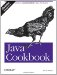# Recipe 5.15 Calculating Trigonometric Functions

#### Problem

You need to compute sine, cosine, and other trigonometric functions.

#### Solution

Use the trig functions in java.lang.Math . Like java.lang.Math.random( ), all the methods of the Math class are static, so no Math instance is necessary. This makes sense, as none of these computations maintains any state. Note that the arguments for trigonometric functions are in radians, not in degrees. Here is a program that computes a few (mostly) trigonometric values and displays the values of e and PI that are available in the math library:

`// Trig.java  System.out.println("Java's PI is " + Math.PI);  System.out.println("Java's e is " + Math.E);  System.out.println("The cosine of 1.1418 is " + Math.cos(1.1418));`

The java.lang.StrictMath class, introduced in JDK 1.3, is intended to perform the same operations as java.lang.Math but with greater cross-platform repeatability.Java Cookbook, Second Edition
ISBN: 0596007019
EAN: 2147483647
Year: 2003
Pages: 409
Authors: Ian F Darwin

Similar book on Amazon